# How to get the first word in Microsoft Excel

If you come across a situation in which you require only first word in each line of a cell rather than all the character, then you must read this article. The following tutorial will you help you in retrieving only first word through VBA code in excel.

In this article, we will focus on how to keep first word only in each line of a cell separated by semicolon via macro codes.

Question: The data I am working in has sometimes multiple lines in each of the cell & I want to extract the first word only.

You can find original question here

Following is a snapshot of input text (Before Sheet)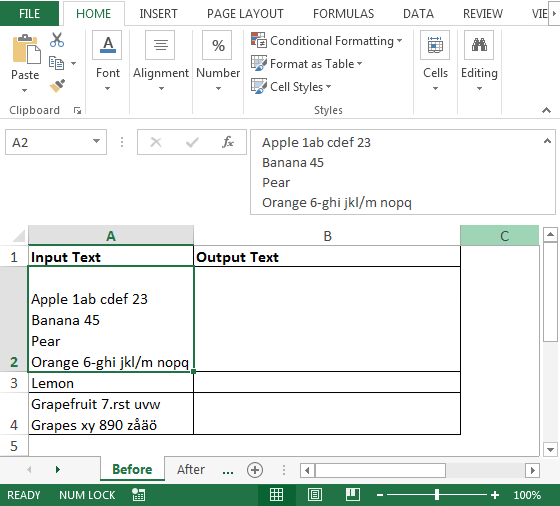Following is a snapshot of input text (After Sheet) in column A & requires output in column B; refer below snapshot: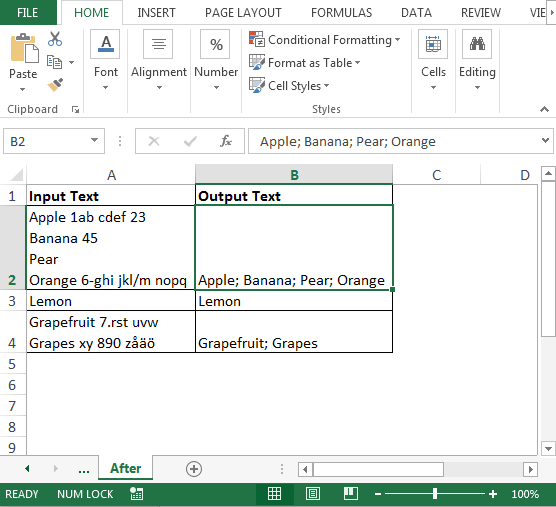To get the code; we need to follow the below steps to launch VB editor

• Click on Developer tab
• From Code group, select Visual Basic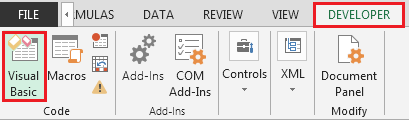• Copy the below code in the standard module

```Function FirstWordOnly(rng As Range)

Dim Arr() As Variant
Dim Count As Integer
Dim i As Integer

Count = Len(rng) - Len(Replace(rng, Chr(10), ""))

If Count > 0 Then
ReDim Arr(0 To Count)
For i = 0 To Count
If i = 0 Then
Arr(i) = Left(rng, InStr(1, rng, " ") - 1)
ElseIf j = 0 Then
j = InStr(1, rng, Chr(10))
Arr(i) = Mid(rng, j + 1, InStr(j, rng, " ") - j)
Else
j = InStr(j + 1, rng, Chr(10))
Arr(i) = Mid(rng, j + 1, InStr(j, rng, " ") - j)
End If
Next

FirstWordOnly = Join(Arr, "; ")
Else
If InStr(1, rng, " ") > 0 Then
FirstWordOnly = Left(rng, InStr(1, rng, " "))
Else
FirstWordOnly = rng
End If

End If

End Function
```

Now, the VBA code is ready for use; we will use the newly created User Defined Function i.e. "FirstWordOnly" in Before Sheet.

• To get the output using UDF in cell B2 the formula will be
• =FirstWordOnly(A2)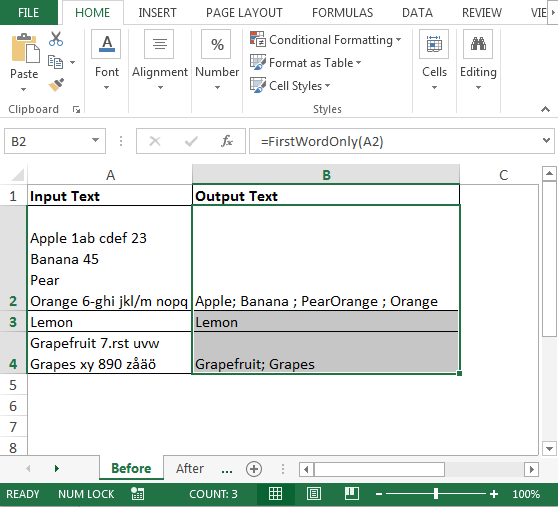Code Explanation:

In the above code, we have used COUNT, LEN, REPLACE, IF, INSTR, MID, CHR, LEFT, JOIN VBA functions.

• ‘Len(rng)’; this will check the length of the cell that we are referring to
• ‘Replace(rng, Chr(10), "")’; The code checks the Chr(10) i.e. line break in each line & then replaces with double quote (nothing)
• ‘Count = Len(rng) - Len(Replace(rng, Chr(10), ""))’; Count will store the difference of length of each line after replacing the line break
• If Count > 0; this will check if the length of Count is greater than zero and if it is found equal to zero i.e. the cell is blank, then the IF condition will not execute the code. To check this, you can use =FirstWordOnly(A5) in cell B5 & it will return 0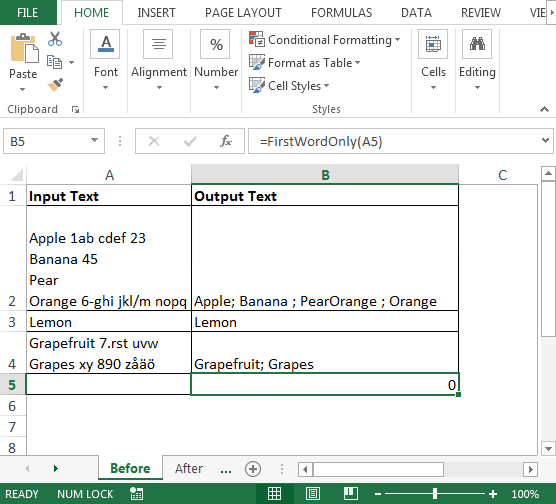• If cell is not blank, then For loop will be used with IF condition and, with LEFT function, we will retrieve the first word
• JOIN function will add semicolon at the end if there are multiple lines in each cell

Conclusion: Using UDF we can remove everything in each line of cell except first word through VBA. This function will work in all the versions from old to new i.e. Microsoft Excel 2003, Microsoft Excel 2007, Microsoft Excel 2010, Microsoft Excel 2013.